Introducing the mecaflux software suite:

Wind turbine propeller modeling in heliciel

Tidal turbine modeling in heliciel Kaplan propeller modeling in heliciel
Wind turbine power and wind speed
The investment made to build our wind turbine will it profitable? After how long the wind turbine will be refunded and will start to return earnings? The wind speed determines the profitability of the project but also the shape and size of our wind turbine propeller.
To build our wind turbine so we have to evaluate a reference speed which will set the operating point of our propeller and a reference speed that will allow us to calculate the average power therefore the profitability of our wind turbine: Nous distinguerons deux vitesses:We distinguish two wind speed: the average speed found at the site, and the operation speed selected as references for the construction of wind turbine.

The wind is not a regular and stable energy flow. It varies depending on the season, time of day, the height at which it is measured, the terrain configuration.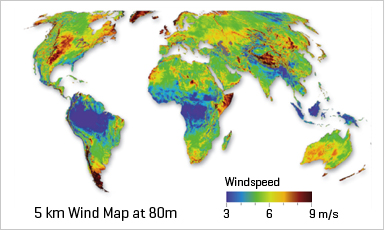What are the factors affecting the change in wind speed ?
• Instant phenomena:gusts. (difficult to assess whether it has no records of weather station)
• Daily phenomena:lthermal winds
• Seaside:
•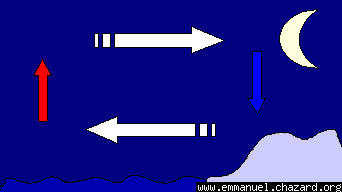• in the mountains: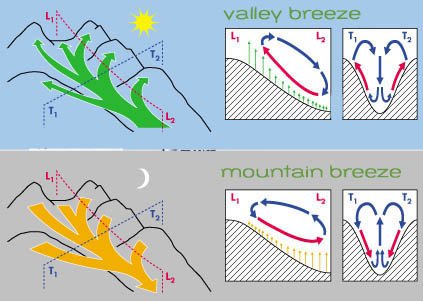• The monthly and seasonal phenomena:
in temperate zones the most frequent winds' s West and South-West, except in the extreme south-east or south-east winds are more frequent Every season is a general direction of winds..
• For the northern hemisphere
• winter : The winds are south west in the north , and north west to the south.
• Spring: The most frequent winds are from the north and west.
• Summer: the schemes are those spring but more established.
• Autumn: the schemes are those winter but more regular.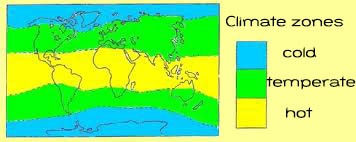• Annual events: Annual data are grouped as wind map. Heliciel proposes a series of wind map by regions and continents:

The vegetation on the ground is also a good indicator of the prevailing winds: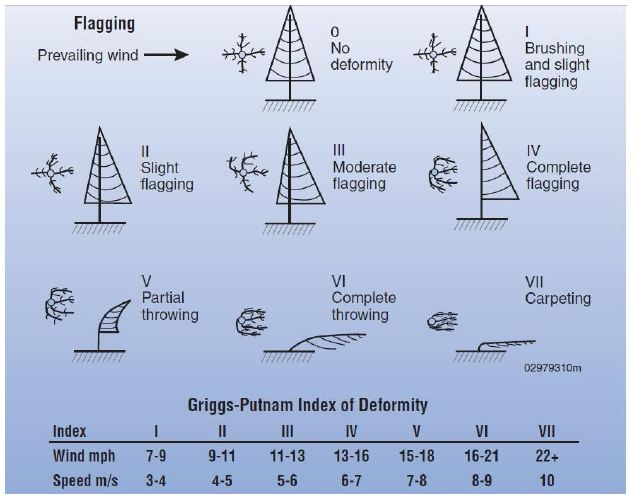• Variations caused by altitude:
The wind maps give the wind at heights from 10 meters. The variation of wind speed with height depends mainly on the shape of the ground. These variations can be represented by a simple law of form: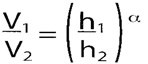The value of the exponent apha is given according to the nature of the ground:

 nature of the ground surface irregularities (cm) exponent apha flat sea ice snow grass short .. 0 to 20 0.08 to 0.12 small irregularities,pasture field crops 20 to 200 0.13 to 0.16 irregular wood sparsely populated areas 1000 to 1500 0.2 to 0.23 very irregular, cities 1000 to 4000 0.25 to 0.4

Example: two-bladed wind wheel diameter of 20 m on a 30 m tower. When the blades are vertical wind speed ratios at the ends of the blades are two extreme values ​​for alpha: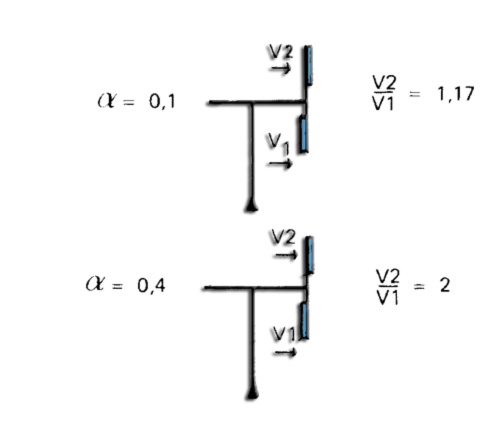This shows that the most interesting sites are those whose land is less rugged and for which the exponent alpha is low. In addition to the loss of wind near the ground, forcing it to build expensive towers, the speed difference between the high and low ends, creates imbalances in the wind turbine. Vibration and further efforts are then generated.
• Variations caused by obstacles and the shape of the site. In the absence of a precise mapping of the wind resource available, you can still locate sites that deserve attention, and make local measurements, more specific and shorter. Some regions are thus naturally blessed by the god Aeolus: Coastal areas, the highlands and mountains, islands, or some plain clear. The corridors of large rivers where the wind can s' rush, are also favored areas, because along the bed of the river, the wind takes a relatively constant direction..
More generally, the most notable sites are those whose roughness is lowest where the wind encounter few barriers. Of course, so we seek rather to install wind turbines in places a little high and clear..
Thus, the interior can harbor particularly interesting sites like the hills, especially if the slope is gentle and gradual. roughness is low, and the flow of air is compressed by the obstacle and thus increases its velocity. The value of that can sometimes almost double: it is the "hill effect":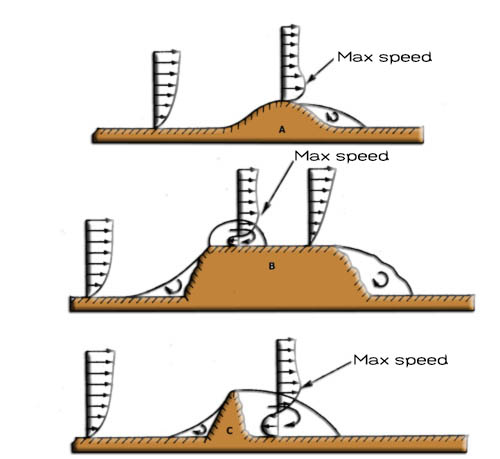• A)Hill with gentle slopes and rounded summit site very favorable
• B)Steep hill slope or cliff top: Site causing severe turbulence (to Avoid)
• C)rocky peak, trees, buildings, houses ... avoid a close installations because there is presence of wind disturbance and downwind.
The average wind speed on the site and the energy generated:

We have collected wind data at the site and we consulted the cards for an average wind speed. A ratio taking into account the height was introduced. how much energy will we produce?

• Determining the Power and Energy of the site:

The kinetic energy of an object is calculated using the mass and velocity:

kinetic energy =0.5 X mass X velocity²

If we consider the second time interval as reference, for a wind moving at constant speed, the mass is the volume of air passing through the area in 1 second X density of air. the mass of Wind passing through a surface S is therefore: Mass = velocity ( m/sec) X air_density(kg/m3) X Surface(m²) .our kinetic energy per second is therefore:

• volume= speed (m/s) X S(m²)
• mass = Volume X density
• kinetic energy = 0.5 X masse X speed² = 0.5 Xspeed X density X speed²=

0.5 X density X S X (speed)³

Particular attention should be given to the calculation of the power:

• Power grows with the cube of the speed, the density of the air and the surface :

P= 0.5 X density X S X (speed)³

p (Watt), density( Kg/m³),speed( m/sec)

This implies two essential things:

1. The density of the air, enters into consideration in the power. This density changes with altitude. (You can change the characteristics of the fluid in héliciel. density varies with the altitude and temperature input. The viscosity is also affected by the selected temperature) At the same wind speed, site altitude, will have a lower potential energy, that at sea level.
2. A small change in speed is enough to cause a significant variation in power: Take the example of a site or the wind is 10 m / sec and another site where the wind is 12 m / sec.
• The speed difference between the two sites is 20% (12/10=1.2)
• the power difference between the two locations (take a density of 1.2 and a surface 1) is :
• P= 0.5 X density X S X (speed)³
• Psite1= 0.5 X 1.2 X1X (10)³ =600
• Psite2= 0.5 X 1.2 X1X (12)³ =1036.8
• power differences=(1036.8/600=1.72) =72%
Doubling the speed multiplies the power by 8!
Returning to our average wind speed recorded for the site. Suppose for simplicity that the average is calculated over two months with two wind speeds: (take a density of 1.2 and a surface 1m² )
• speed in March=10m/s and April speed=20m/s .
• Average wind= (10+20)/2=15 m/s
• Power average of the wind = 0.5 X 1.2 X1X (15)³ = 2025
Now calculate first the March power and power in April and perform the average:
• power in March=0.5 X 1.2 X1X (10)³=600
• power in April=0.5 X 1.2 X1X (20)³ =4800
• Average power= (600+4800)/2=2700
We find that average power is stronger than the power of the average! This implies that an average wind to make a forecast of power generates under power assessment. More wind data are averaged less accurate we are. To accurately predict the power and energy that we can extract of the site,it is better to make the sum of power generated, to different speeds measured by the shortest time possible. Weather data are valuable and monthly statements are useful. Even with weather data values ​​can vary from 25% a year over the other. The accuracy of predictions should be considered with caution. A windmill farm will have good and bad years.
However, mathematical methods are used to assess the power from the average speeds:Weibull distribution or distribution Rayleight ...
Incidentally, if we consider a surface of 1m ² for power calculations on the site, it greatly simplifies the calculations, from now on we will refer to the power to 1m ². Power per square meter is the power density of the site. We will see that this notion is doubly convenient because in addition to simplifying the power equation, it allows us to directly find the power of the wind turbine by multiplying the power density (power per 1m ²) by the area swept by the wind turbine!

To evaluate the profitability of a wind turbine project: we will compare the money invested and the money generated:

• For the money invested, an inventory of materials and workmanship (not forgetting maintenance) will be done.
• For money product we multiply the energy generated by the sale price of energy. Conversion between average power to the site and energy generated needs to be made:
• Power on the site will be reduced by the efficiency of the wind turbine. The performance of the wind turbine, we have seen on the wind turbine energy page, will allow us to convert between 14 and 30% of the power of the site in the best case. Example: If the average power of our site is: "150 w / m" and our wind turbine has an efficiency of 20%, so we will have a power output per m² of wind turbine swept area of: 150 X 0.2 = 30 w =0.03Kw
• The product sold is not power, but the energy.The sales unit of electrical energy is Kw / hour. 1 kilowatt / h is produced by delivering a power of 1kw for an hour. If our wind turbine product 0.03Kw averaged over the year per m², taking into account the fact that a year contains 8,760 hours, energy production over the year will be:
8760 h X 0.03Kw = 262.8Kw/h per m²
Kwh is charged 12.8 cents (average value inLos Angeles 2013) . L'éolienne fournira donc une économie de 263X0.13=34.19 \$ par m² per year on this site. If a wind turbine, with blades of 1 meter scanning a surface of 3.14 m², is located on the site it will produce a saving of 3.14 X 34.19=107\$per year.

The nominal wind speed of operation of our wind turbine:
As we have seen above, the average wind speed is found on maps or according the measures on the ground. Attention will be paid to the frequency of wind. The choice of the nominal wind speed and the speed of rotation according to:
• Specifications generator,
• the power captured
• and wind speed considered as speed reference.

Once the blade has been built twist can not be changed. A variation of the pitch is possible but the twist should have been optimized for wind speed (rated wind speed) and rotation (operating point) chosen.

bibliographic reference